# SAT Math Multiple Choice Question 789: Answer and Explanation

### Test Information

Question: 789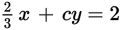9. If the slope of the equation shown above is 6, what is the value of c?

• A. –4
• B. -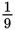• C.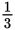• D. 4

Explanation:

B

Difficulty: Medium

Category: Heart of Algebra / Linear Equations

Strategic Advice: Don't overthink a question like this. It is asking about slope and you have an equation, so write the equation in slope-intercept form and see where that takes you.

Getting to the Answer: When an equation is written in slope-intercept form (y = mx + b), m is the slope of the line. Rewrite the given equation in this form by subtracting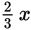from both sides and then dividing both sides by c: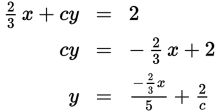Now, set the coefficient of x equal to the given slope (6) and solve for c. The coefficient is a fraction, so write 6 as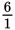and cross-multiply: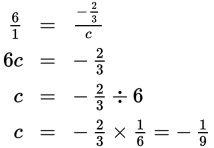The value of c is -, which is (B).